# Trigonometry : Angles

## Example Questions

← Previous 1 3 4 5 6 7 8

### Example Question #1 : Angles

Determine the value for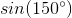in radical form.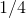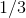Explanation:

According to the unit circle, an angle of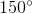is similar to an angle of.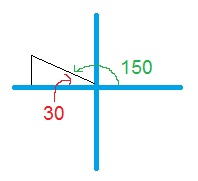Therefore: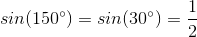### Example Question #1 : Angles

What is the relationship between angles 1 and 2?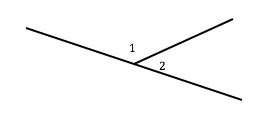equal

horizontal

vertical

supplementary

complimentary

supplementary

Explanation:

These angles are supplementary, as the sum of the angles is 180o.

### Example Question #1 : Angles

What angle is supplementary to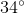?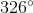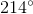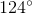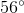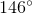Explanation:

Two angles that are supplementary add up to. For our problem, that means that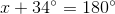.

Subtractfrom both sides.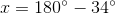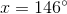### Example Question #1 : Angles

Find the complementary angle to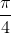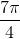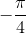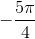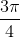Explanation:

Two angles that are complementary must add to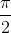, therefore we subtract the given angle fromas follows: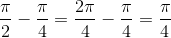### Example Question #2 : Complementary And Supplementary Angles

Find the complementary angle to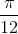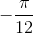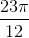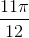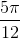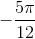Explanation:

Two angles are complementary if their sum is, therefore we subtract the given angle fromas follows: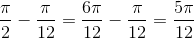### Example Question #3 : Complementary And Supplementary Angles

Find the angle that is supplementary to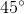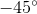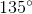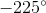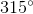Explanation:

Two angles are supplementary if their sum is, therefore we subtract the given angle fromas follows: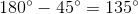### Example Question #1 : Complementary And Supplementary Angles

Find the angle that is supplementary to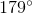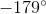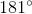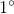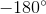Explanation:

Two angles are supplementary if their sum is, therefore we subtract the given angle fromas follows: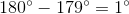### Example Question #1 : Complementary And Supplementary Angles

Find the angle that is supplementary to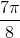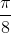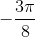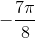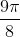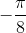Explanation:

If two angles are supplementary their sum is, therefore we subtract the given angle fromas follows: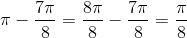### Example Question #2 : Complementary And Supplementary Angles

Find the complementary angle to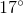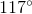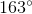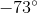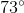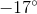Explanation:

In order for two angles to be complementary their sum must be, therefore the complementary angle can be found by subtracting the given angle from: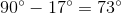### Example Question #10 : Angles

Determine the complementary angle to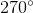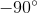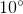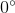Explanation:

The sum of two complementary angles must be, therefore in order to determine the complementary angle ofwe simply subtract fromas follows: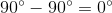← Previous 1 3 4 5 6 7 8

### All Trigonometry Resources